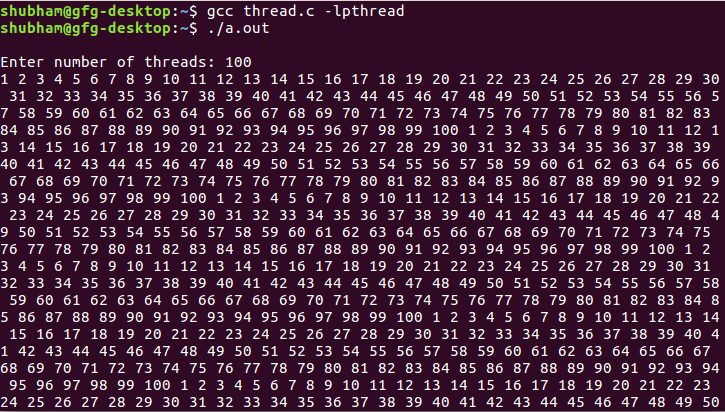Open in App
Not now

# Print numbers in sequence using thread synchronization

• Difficulty Level : Hard
• Last Updated : 23 Dec, 2021

The problem is to synchronize n number of threads using pthread library. The idea is take thread count and print 1 in first thread, print 2 in second thread, print 3 in third thread, ….. print n in nth thread and again repeat from thread 1 infinitely.

Examples :

```Input : Thread count
Output : 1 2 3 ... thread count 1 2 3 ... thread
count 1 2 3 ... thread count ....

Input : 5
Output : 1 2 3 4 5 1 2 3 4 5 1 2 3 4 5 1 2 3 4 5
1 2 3 4 5 1 2 3 4 5 ....```

Below is the implementation:

## CPP

 `// C code to synchronize threads ` `#include ` `#include ` `#include ` `#include ` ` `  `pthread_mutex_t mutex = PTHREAD_MUTEX_INITIALIZER; ` `pthread_cond_t* cond = NULL; ` ` `  `int` `threads; ` `volatile` `int` `cnt = 0; ` ` `  `// function to synchronize threads ` `void``* foo(``void``* arg) ` `{ ` `    ``// turn is a basically to identify a thread ` `    ``int` `turn = *(``int``*)arg; ` ` `  `    ``while` `(1) { ` `        ``pthread_mutex_lock(&mutex); ` ` `  `        ``// cnt is used to determine which thread should ` `        ``// enter into critical section(printf() statement) ` `        ``if` `(turn != cnt) { ` ` `  `            ``// put all thread except one thread in waiting ` `            ``// state ` `            ``pthread_cond_wait(&cond[turn], &mutex); ` `        ``} ` ` `  `        ``// it's a time to print turn can have ` `        ``// values starting from 0. Hence + 1 ` `        ``printf``(``"%d "``, turn + 1); ` ` `  `        ``// determine which thread need to be scheduled now ` `        ``if` `(cnt < threads - 1) { ` `            ``cnt++; ` `        ``} ` `        ``else` `{ ` `            ``cnt = 0; ` `        ``} ` ` `  `        ``// wake up next thread ` `        ``pthread_cond_signal(&cond[cnt]); ` `        ``pthread_mutex_unlock(&mutex); ` `    ``} ` ` `  `    ``return` `NULL; ` `} ` ` `  `// Driver code ` `int` `main() ` `{ ` `    ``pthread_t* tid; ` `    ``volatile` `int` `i; ` `    ``int``* arr; ` ` `  `    ``printf``(``"\nEnter number of threads: "``); ` `    ``scanf``(``"%d"``, &threads); ` ` `  `    ``// allocate memory to cond (conditional variable), ` `    ``// thread id's and array of size threads ` `    ``cond = (pthread_cond_t*)``malloc``(``sizeof``(pthread_cond_t) ` `                                   ``* threads); ` `    ``tid = (pthread_t*)``malloc``(``sizeof``(pthread_t) * threads); ` `    ``arr = (``int``*)``malloc``(``sizeof``(``int``) * threads); ` ` `  `    ``// Initialize cond (conditional variable) ` `    ``for` `(``int` `i = 0; i < threads; i++) { ` `        ``if` `(pthread_cond_init(&cond[i], NULL) != 0) { ` `            ``perror``(``"pthread_cond_init() error"``); ` `            ``exit``(1); ` `        ``} ` `    ``} ` ` `  `    ``// create threads ` `    ``for` `(i = 0; i < threads; i++) { ` `        ``arr[i] = i; ` `        ``pthread_create(&tid[i], NULL, foo, (``void``*)&arr[i]); ` `    ``} ` ` `  `    ``// waiting for thread ` `    ``for` `(i = 0; i < threads; i++) { ` `        ``pthread_join(tid[i], NULL); ` `    ``} ` ` `  `    ``return` `0; ` `}`

Output :My Personal Notes arrow_drop_up
Related Articles